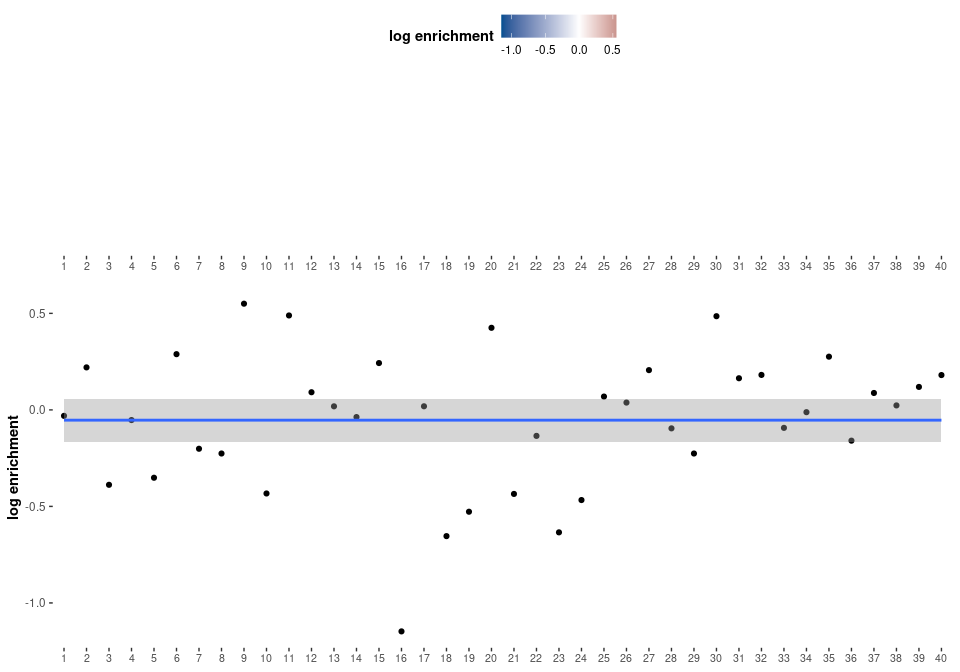# Spectrum Motif Analysis (SPMA)

#### 2023-04-25

In order to investigate how RBP targets are distributed across a spectrum of transcripts (e.g., all transcripts of a platform, ordered by fold change), Spectrum Motif Analysis visualizes the distribution of putative RBP binding sites across all transcripts.

# Analysis

library(transite)

Load example data set from transite package, a data frame with 1000 rows and the following columns:

• refseq: RefSeq identifiers
• value: signal-to-noise ratios between treatment and control samples (sorting criterion)
• seq_type: specifying the type of sequence in seq column; either 3’-UTR, 5’-UTR, or entire mRNAs (including 5’-UTRs, coding regions, and 3’-UTRs)
• seq: sequence
background_df <- transite:::ge$background_df Sort sequences (i.e., transcripts) by ascending signal-to-noise ratio. Transcripts upregulated in control samples are at the beginning of list, transcripts upregulated in treatment samples at the end. background_df <- dplyr::arrange(background_df, value) The DNA sequences in the data frame are converted to RNA sequences. Motifs in the Transite motif database are RNA-based. background_set <- gsub("T", "U", background_df$seq)

Prepare named character vector of presorted sequences for run_matrix_spma. The function expects a character vector containing sequences, sorted in a meaningful way, named according to the following format: [REFSEQ]|[SEQ_TYPE]

The name is only important if results are cached. It is used as the key in a dictionary that stores the number of putative binding sites for each RefSeq identifier and sequence type.

names(background_set) <- paste0(background_df$refseq, "|", background_df$seq_type)

In this example we limit our analysis to an arbitrarily selected motif in the motif database in order to reduce run-time.

motif_db <- get_motif_by_id("M178_0.6")

Matrix-based SPMA is executed:

results <- run_matrix_spma(background_set, motifs = motif_db, cache = FALSE)
## Warning in min(diff(unique_loc)): no non-missing arguments to min; returning
## Inf
# Usually, all motifs are included in the analysis and results are cached to make subsequent analyses more efficient.
# results <- run_matrix_spma(background_set)

# Results

Matrix-based SPMA returns a number of result objects, combined in a list with the following components:

• foreground_scores
• background_scores
• enrichment_dfs
• spectrum_info_df
• spectrum_plots
• classifier_scores

In the following, we plot the spectrum plot, showing how putative binding sites are distributed across the spectrum of transcripts. (spectrum_plots).

In addition, we show statistics describing the spectrum plot (spectrum_info_df).

####BRUNOL6, CELF3 (M178_0.6)

Spectrum plot with polynomial regression:Classification:

spectrum classification: random (0 out of 3 criteria met)

Property Value Threshold
adjusted $$R^2$$ 0 $$\geq 0.4$$
polynomial degree 0 $$\geq 1$$
slope 0 $$\neq 0$$
unadjusted p-value estimate of consistency score 0.9428114 $$< 0.000005$$
number of significant bins 0 $$\geq 4$$

Visual inspection of the spectrum plot as well as the classification and the underlying properties suggest that the putative binding sites of this particular RNA-binding protein are distributed in a random fashion across the spectrum of transcripts. There is no evidence that this RNA-binding protein is involved in modulating gene expression changes between treatment and control group.